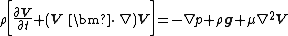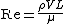Navier-Stokes equation

Navier-Stokes equation

A partial differential equation which describes the conservation of linear momentum for a linearly viscous (newtonian), incompressible fluid flow. In vector form, this relation is written as Eq. (1),

(1)where &rgr; is fluid density, V is fluid velocity, p is fluid pressure, g is the gravitational acceleration, μ is fluid viscosity, ∇ is the del or grad operator, and ∇2 is the laplacian operator. The equation is named after its two principal developers, French engineer C. L. M. H. Navier (1823) and Irish scientist George G. Stokes (1845). When coupled with the conservation of mass relation, ∇ · V = 0, Eq. (1) can be solved for the space-time distribution of V and p in a given region of viscous fluid flow. Typical boundary conditions are (1) the knowledge of the velocity and pressure in the far field, and (2) the no-slip condition at solid surfaces (fluid velocity equals solid velocity). See Newtonian fluid, Viscosity

Equation (1) correctly models the continuum behavior of all newtonian fluids, including the disorderly fluctuating motion known as turbulence. However, since the left-hand side is highly nonlinear, only a few score of exact solutions are known, mostly for simple geometries. The primary dimensionless parameter which governs Eq. (1) is the Reynolds number, given by Eq. (2),

(2)where L is a characteristic body dimension. For small Re 1, Eq. (1) can be simplified by neglecting the left-hand side, resulting in a linear approximation called Stokes flow, or creeping flow, for which many solutions are known. See Creeping flow, Reynolds number

For large Re 1, viscous effects are often confined to a thin boundary layer near solid surfaces, with the remaining flow being nearly inviscid. See Boundary-layer flow

References in periodicals archive ?
High-resolutions for incompressible Navier-Stokes equation and a multi-grid method, J.
In case of an incompressible Newtonian viscous fluid, continuity equation and Navier-Stokes equation are as follows:
Equation (1) is the Navier-Stokes equation that describes the flow of incompressible fluids.
The solution of the fluid side is based on the continuity equation and the Navier-Stokes equation.
Non-Newtonian fluids are the most widely occurring fluids in industry and engineering and its governing equations are highly nonlinear as compared to the Navier-Stokes equation.
Soltanov: On some modification Navier-Stokes equation, Nonlinear Anal.
Streamline upwind/Petrov-Galerkin formulation for convection dominated ows with particular emphasis on the incompressible Navier-Stokes equation.
The first exact solution of the Navier-stokes equation was given by Stokes  which is concerned with the flow of a viscous incompressible fluid past an infinite horizontal plate oscillating in its own plane in an infinite mass of stationary fluid.
The professor of the mathematical analysis department of the Kyrgyz National University, Taalaibek Omurov, announced he found the solution to the Navier-Stokes Equation.
FFD, developed by Stam (1999) for computer flow visualization, can efficiently solve the Navier-Stokes equation (Equation 1), energy equation (Equation 2), and species transport equation (Equation 3):
The equations for the flow inside the drops are given by the equation of continuity for an incompressible fluid and the Navier-Stokes equation within the quasistatic approximation, so that
The electric field (E = - [nabla][psi] inside the capillary is coupled to Navier-Stokes equation to give the average fluid velocity,

Site: Follow: Share:
Open / Close